# Math Olympiad Test: Circles- 1 - Class 10

Test Description

## 10 Questions MCQ Test Olympiad Preparation for Class 10 - Math Olympiad Test: Circles- 1

Math Olympiad Test: Circles- 1 for Class 10 2023 is part of Olympiad Preparation for Class 10 preparation. The Math Olympiad Test: Circles- 1 questions and answers have been prepared according to the Class 10 exam syllabus.The Math Olympiad Test: Circles- 1 MCQs are made for Class 10 2023 Exam. Find important definitions, questions, notes, meanings, examples, exercises, MCQs and online tests for Math Olympiad Test: Circles- 1 below.
 1 Crore+ students have signed up on EduRev. Have you?
Math Olympiad Test: Circles- 1 - Question 1

### Two chords AB and CD of a circle intersect at an external point P as shown in figure. If AB = 8 cm, BP = 10 cm, PD = 12 cm. What is length of CP?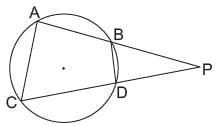Detailed Solution for Math Olympiad Test: Circles- 1 - Question 1

For any external point P,
AP × BP = PD × PC
⇒ (AB + BP) × BP = 12 × PC
⇒ (8 +10) × 10 = 12 × PC
⇒ PC = 180/12 = 15 cm.

Math Olympiad Test: Circles- 1 - Question 2

### What is the length of tangent segment PT to a circle whose centre is at O. OP = 17 cm and OT = 8 cm?

Detailed Solution for Math Olympiad Test: Circles- 1 - Question 2

In DOPT,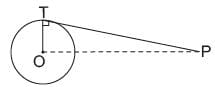OT2 + PT2 = OP2
⇒ PT =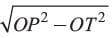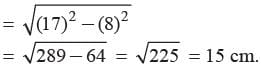Math Olympiad Test: Circles- 1 - Question 3

### The side of a square is 10 cm. What is the area of circumscribed circle?

Detailed Solution for Math Olympiad Test: Circles- 1 - Question 3

Diameter of the circumscribed circle
= diagonal of the square
= 10√2cm
Radius =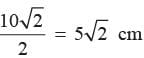Area of circumscribed circle = πr2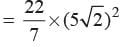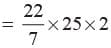= 157 cm2.

Math Olympiad Test: Circles- 1 - Question 4

What is the area of circle in which the difference between the radius and circumference of the circle be 37 cm?

Detailed Solution for Math Olympiad Test: Circles- 1 - Question 4

Let r be the radius of the circle
2πr - r = 37
⇒ r (2π - 1) = 37
⇒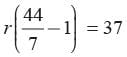⇒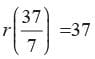⇒ r = 7 cm

Area = πr2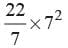= 22 × 7 = 154 cm2.

Math Olympiad Test: Circles- 1 - Question 5

How the tangent at any point of a circle and radius through the point is related?

Detailed Solution for Math Olympiad Test: Circles- 1 - Question 5

The tangent at any point of a circle and the radius through this point are perpendicular to each other as shown below: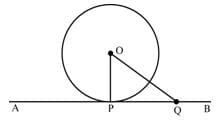In the figure above,
∠OPA +∠OPQ = 180 ........(1) [Linear pair angle]
Also, ∠OPA = ∠OPQ ......(2)
∴ ∠OPA +∠OPQ = 180
2 ×∠OPQ = 180
∠OPQ = 90
Hence, OP is perpendicular to PQ ⇒ OP is perpendicular to AB.

Math Olympiad Test: Circles- 1 - Question 6

The diameter of the front and rear wheels of a tractor are 80 cm and 200 cm respectively. What are the number of revolution that a rear wheel makes to cover the distance which the front wheel covers in 800 revolutions?

Detailed Solution for Math Olympiad Test: Circles- 1 - Question 6

Radius of front of wheel = 40 cm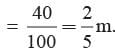Circumference =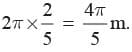Distance moved by it in 800 revolutions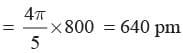Circumference of real wheel = 2π × 1 = 2π m.
No. of revolutions =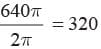Math Olympiad Test: Circles- 1 - Question 7

A copper wire when bent in the form of a square encloses an area of 484 cm2. The same wire is now bent in the form of a circle. Find the area enclosed by the circle.

Detailed Solution for Math Olympiad Test: Circles- 1 - Question 7

Area of square = 484
Side of square =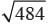= 22 cm
⇒ 2πr= 22 × 4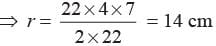Area of circle = πr2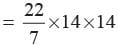= 44 × 14
= 616 cm2.

Math Olympiad Test: Circles- 1 - Question 8

A race track is in the form of a ring whose inner and outer circumference are 437 m and 50 m respectively. Find the area of the track.

Detailed Solution for Math Olympiad Test: Circles- 1 - Question 8

Let R be the radius of outer circle and r be the radius of inner circle
2πR = 503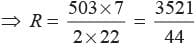and 2πr = 437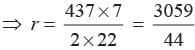Width of the track = R - r =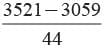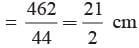Area of the track = π(R2 - r2)
= π(R + r) (R - r)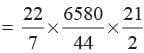= 4935 m2

Math Olympiad Test: Circles- 1 - Question 9

A square park has each side of 100 m. At each comer of the park, there is a flower bed in the form of a quadrant inside the park of radius 14 m. What is the area of remaining part?

Detailed Solution for Math Olympiad Test: Circles- 1 - Question 9

Let A be the area of each quadrant of the circle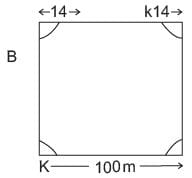Radius of circel = 14 cm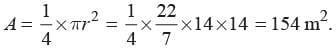Area of 4 quadrants = 4 ×154 = 616 m2 Area of square park = (100)2 = 10000 m2
Area of remaining part = 10000 - 616
= 9384 m2

Math Olympiad Test: Circles- 1 - Question 10

If the area of a sector of a circle is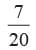of the area of the circle, then what is the sector angle of the circle?

Detailed Solution for Math Olympiad Test: Circles- 1 - Question 10

Area of the sector =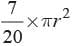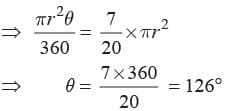## Olympiad Preparation for Class 10

11 videos|36 docs|201 tests